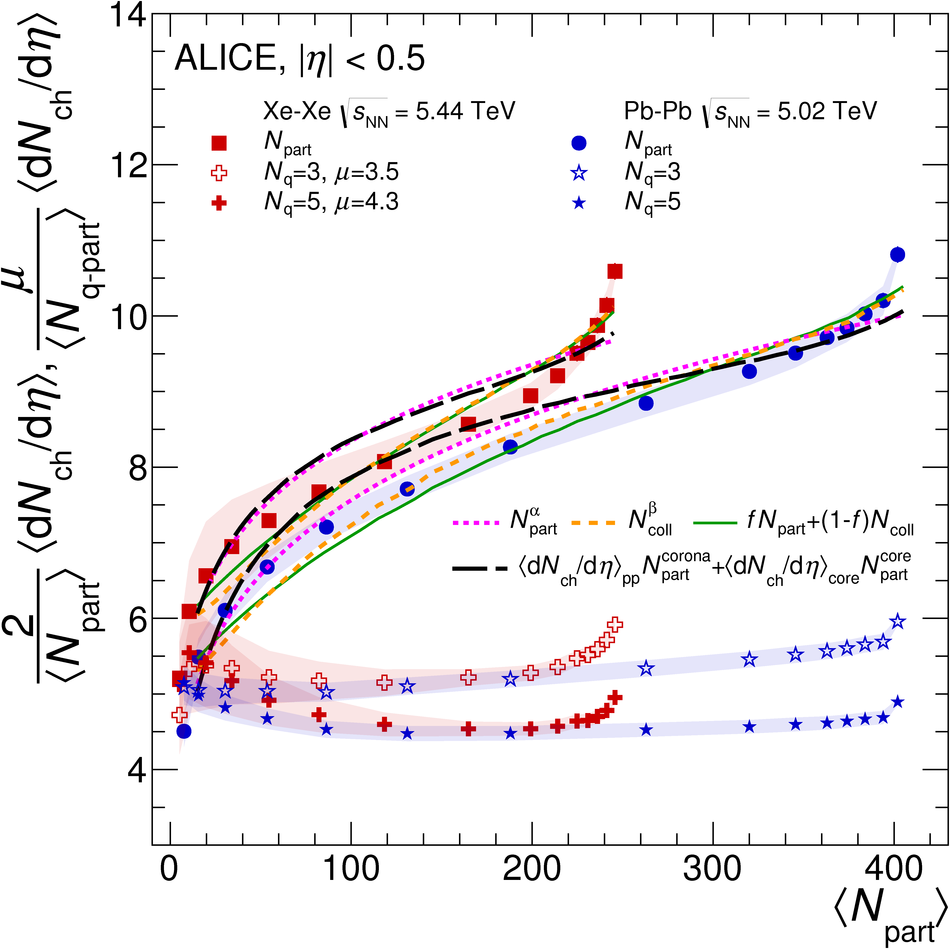# Centrality and pseudorapidity dependence of the charged-particle multiplicity density in Xe-Xe collisions at $\sqrt{s_{\rm NN}}$ = 5.44 TeV

In this Letter, the ALICE Collaboration presents the first measurements of the charged-particle multiplicity density, $\rm{d}N_{\rm{ch}}/\rm{d}\eta$, and total charged-particle multiplicity, $N_{\rm{ch}}^{\rm{tot}}$, in Xe-Xe collisions at a centre-of-mass energy per nucleon--nucleon pair of $\sqrt{s_{\rm NN}}$ = 5.44 TeV. The measurements are performed as a function of collision centrality over a wide pseudorapidity range of $-3.5 <~ \eta <~ 5$. The values of $\rm{d}N_{\rm{ch}}/\rm{d}\eta$ at mid-rapidity and $N_{\rm{ch}}^{\rm{tot}}$ for central collisions, normalised to the number of nucleons participating in the collision ($N_{\rm{part}}$) as a function of $\sqrt{s_{\rm NN}}$, follow the trends established in previous heavy-ion measurements. The same quantities are also found to increase as a function of $N_{\rm{part}}$, and up to the 5% most central collisions the trends are the same as the ones observed in Pb-Pb at a similar energy. For more central collisions, the Xe-Xe scaled multiplicities exceed those in Pb-Pb for a similar $N_{\rm{part}}$. The results are compared to phenomenological models and theoretical calculations based on different mechanisms for particle production in nuclear collisions. All considered models describe the data reasonably well within 15%.

Figures

## Figure 1

 Charged--particle pseudorapidity density for 12 centrality classes over a broad $\eta$ range in \XeXe\ collisions at \snn~=~\unit[5.44]{TeV}. Boxes around the points reflect the total systematic uncertainties, while the filled squares on the right reflect the normalisation uncertainty from the centrality determination Statistical errors are negligible The reflection (open circles) of the $3.5< \eta< 5$ values around $\eta=0$ is also shown The lines correspond to fits to a gaussian distribution in rapidity multiplied by an effective Jacobian of transformation from $\eta$ to $y$.## Figure 2

 Values of $\dNdetape$ (top) and $\frac{2}{\langle N_\mathrm{part}\rangle} N^\mathrm{tot}_\mathrm{ch}$ (bottom) for the 5\% most central \XeXe\ collisions compared to previous measurements in \PbPb\ and \AuAu\ as a function of \snn, as well as for inelastic \pp, \ppbar\ and non-single diffractive pA and dA collisions The lines are power law fits to the data, excluding \XeXe\ results The central \PbPb\ measurements from CMS and ATLAS at \unit[2.76]{TeV} have been shifted horizontally for clarity.## Figure 3

 The \dNdetape\ (top) and $\frac{2}{\langle N_\mathrm{part}\rangle} N^\mathrm{tot}_\mathrm{ch}$ (bottom) for \XeXe\ collisions at \snn~=~\unit[5.44]{TeV} as a function of \avNpart The error bars indicate the point-to-point centrality-dependent uncertainties whereas the shaded band shows the correlated contributions. Also shown in the figure is the result from inelastic \pp\ at \s~=~\unit[5.02]{TeV} as well as non-single diffractive \pPb\ collisions~ and \PbPb\ collisions at \snn~=~\unit[5.02]{TeV}~ Note that \PbPb\ data at \snn~=~\unit[5.02]{TeV} were re-analysed in narrower centrality classes Data from lower energies at \snn~=~\unit[2.76]{TeV} and \unit{GeV} are shown for comparison.## Figure 4

 The \dNdetape\ (top) and $\frac{2}{\langle N_\mathrm{part}\rangle} N^\mathrm{tot}_\mathrm{ch}$ (bottom) for \XeXe\ collisions at \snn~=~\unit[5.44]{TeV} as a function of $(\avNpart-2)/(2A)$.## Figure 5

 The $\dNdetape$ for \XeXe\ collisions at \snn~=~\unit[5.44]{TeV} and \PbPb\ collisions at \snn~=~\unit[5.02]{TeV}~, as a function of $\langle N_{\mathrm{part}} \rangle$. The \PbPb\ data are fitted with various parameterisations of \Npart\ and \Ncoll, calculated with the Glauber model The same functions, with the values of the parameters from the Pb--Pb fit, are used for the \XeXe\ data Also shown is \dNdeta\ per participant quark, \Ncpart, calculated with the effective wounded constituent quarks model~, as a function of \avNpart. The number of participant quarks \Ncpart\ is normalised by the average number of participant quarks in \pp\ collisions, $\mu$.## Figure 6

 The $\dNdetape$ for \XeXe\ collisions at \snn~=~\unit[5.44]{TeV} as a function of $\langle N_{\mathrm{part}} \rangle$ compared to model predictions . The bottom panel shows the ratio of the models to the data The shaded band around the points reflects the correlated systematic uncertainties.## Figure 7

 Comparison of \dndeta\ as a function of $\eta$ in the 0--5\% central class to model predictions. The bottom panel shows the ratio of the models to the data. Boxes around the points reflect the total uncorrelated systematic uncertainties.